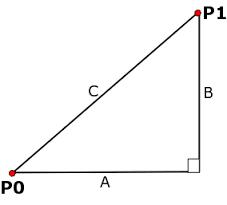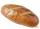# Euclidean distance

Calculate the Euclidean distance between shops A, B, and C, where:
A 45 0.05
B 60 0.05
C 52 0.09

The first figure is the weight in grams of bread, and the second figure is the USD price.

|AB| =  15
|BC| =  8.0001
|AC| =  7.00011

### Step-by-step explanation:

$\mathrm{\mid }AB\mathrm{\mid }=\sqrt{\left(45-60{\right)}^{2}+\left(0.05-0.05{\right)}^{2}}\doteq 15$
$\mathrm{\mid }BC\mathrm{\mid }=\sqrt{\left(60-52{\right)}^{2}+\left(0.05-0.09{\right)}^{2}}\doteq 8.0001$
$\mathrm{\mid }AC\mathrm{\mid }=\sqrt{\left(45-52{\right)}^{2}+\left(0.05-0.09{\right)}^{2}}\doteq 7.00011$Did you find an error or inaccuracy? Feel free to write us. Thank you!Tips to related online calculators
Pythagorean theorem is the base for the right triangle calculator.

#### You need to know the following knowledge to solve this word math problem:

We encourage you to watch this tutorial video on this math problem:

## Related math problems and questions:

• Plane IIA plane flew 50 km on a bearing 63degrees20, and the flew on a bearing 153degrees20 for 140km. Find the distance between the starting point and the ending point.
• Without Euclid lawsRight triangle ABC with right angle at the C has a=14 and hypotenuse c=26. Calculate the height h of this triangle without the use of Euclidean laws.
• Reverse Pythagorean theoremGiven are lengths of the sides of the triangles. Decide which one is rectangular: Δ ABC: 77 dm, 85 dm, 36 dm ... Δ DEF: 55 dm, 82 dm, 61 dm ... Δ GHI: 24 mm, 25 mm, 7 mm ... Δ JKL: 32 dm, 51 dm, 82 dm ... Δ MNO: 51 dm, 45 dm,
• ConcentrationHow many grams of 65% and 50% acid is to be mixed to give 240 g acid with a concentration of 60%?
• Euklid4The legs of a right triangle have dimensions 244 m and 246 m. Calculate the length of the hypotenuse and the height of this right triangle.
• BearingA plane flew 50 km on a bearing 63°20' and the flew on a bearing 153°20' for 140km. Find the distance between the starting point and the ending point.
• Tea blendTea blends are maked from two kinds of tea. In standard tea mixture are two teas in the ratio 1:3 and 40 g costs 42 CZK. In the premium tea mixture are weighing two teas in the ratio 1:1 and 50 grams costs 60 CZK. How much cost 10 grams of more expensive
• Altitude anglesCities A, B, C lie in one elevation plane. C is 50 km east of B, B is north of A. C is deviated by 50° from A. The plane flies around places A, B, C at the same altitude. When the aircraft is flying around B, its altitude angle to A is 12°. Find the altit
• Proof PTCan you easily prove Pythagoras theorem using Euclidean theorems? If so, do it.
• An observerAn observer standing west of the tower sees its top at an altitude angle of 45 degrees. After moving 50 meters to the south, he sees its top at an altitude angle of 30 degrees. How tall is the tower?
• Resultant forceCalculate mathematically and graphically the resultant of three forces with a common center if: F1 = 50 kN α1 = 30° F2 = 40 kN α2 = 45° F3 = 40 kN α3 = 25°
• Isosceles triangle 9Given an isosceles triangle ABC where AB= AC. The perimeter is 64cm, and the altitude is 24cm. Find the area of the isosceles triangle.
• TrianglesFind out whether given sizes of the angles can be interior angles of a triangle: a) 23°10',84°30',72°20' b) 90°,41°33',48°37' c) 14°51',90°,75°49' d) 58°58',59°59',60°3'5 of the same bread has the same weight as three bread and 4 kg of fruit. What weight has one bread?The hypotenuse of a right triangle is 41 and the sum of legs is 49. Calculate the length of its legs.Determine the dimensions of the cuboid, if diagonal long 53 dm has an angle with one edge 42° and with another edge 64°.Right triangle BTG has hypotenuse g=117 m and altitude to g is 54 m. How long are hypotenuse segments?